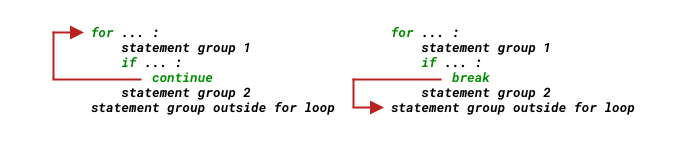Article Outline

# 流程控制

``````for n in range(2, 100):
if n == 2:
print(n)
continue
for i in range(2, n):
if (n % i) == 0:
break
else:
print(n)``````

## if 语句

`if` 语句的最简单构成是这样 —— 注意第 1 行末尾的冒号 `:` 和第 2 行的缩进：

``````if expression:
statements``````

``````import random
r = random.randrange(1, 1000)

if r % 2 == 0:
print(f'{r} is even.')``````
``372 is even.``

``````if expression:
statements_for_True
else:
statements_for_False``````

``````import random
r = random.randrange(1, 1000)

if r % 2 == 0:
print(f'{r} is even.')
else:
print(f'{r} is odd.')``````
``945 is odd.``

``````if expression_1:
statements_for_expression_1_True

elif expression_2:
statements_for_expression_2_True

elif expression_3:
statements_for_expression_3_True

elif expression_...:
statements_for_expression_..._True``````

Python 用 `elif` 处理这种多情况分支，相当于其它编程语言中使用 `switch` 或者 `case`……

`elif``else if` 的缩写，作用相同。

``````import random
r = random.randrange(2, 13)

if r == 7:
print('Draw!')
elif r < 7:
print('Small!')
elif r > 7:
print('Big!')``````
``Big!``

``````import random
r = random.randrange(0, 13) # 生成的随机数应该从 0 开始了；

if r == 7:
print('Draw!')
elif r >= 2 and r < 7:      # 如果这里直接写 elif r < 7:，那么，else: 那一部分永远不会被执行……
print('Small!')
elif r > 7:
print('Big!')
else:
print('Not valid!')``````
``Small!``

## for 循环

Python 语言中，`for` 循环不使用其它语言中那样的计数器，取而代之的是 `range()` 这个我称其为 “整数等差数列生成器” 的函数。

``````for( a = 0; a < 10; a = a + 1 ){
printf("value of a: %d\n", a);
}``````

``````for a in range(10):
print(f'value of a: {a}') #每次 a 的值都不同，从 0 递增至 9``````
``````value of a: 0
value of a: 1
value of a: 2
value of a: 3
value of a: 4
value of a: 5
value of a: 6
value of a: 7
value of a: 8
value of a: 9``````

### range() 函数

`range()` 是个内建函数，它的文档是这样写的：

range(stop)

range(start, stop[, step])

``````print(range(10))
print(list(range(10))) # 将 range(10) 转换成 list，以便清楚看到其内容。``````
``````range(0, 10)
[0, 1, 2, 3, 4, 5, 6, 7, 8, 9]``````

`start` 参数的默认值是 `0`。如需指定起点，那么得给 `range()` 传递两个参数，比如，`range(2, 13)`……

``print(list(range(2, 13)))``
``[2, 3, 4, 5, 6, 7, 8, 9, 10, 11, 12]``

``````for i in range(1, 10, 2):
print(i)``````
``````1
3
5
7
9``````

``````a = list(range(0, -10, -1))
print(a)``````
``[0, -1, -2, -3, -4, -5, -6, -7, -8, -9]``

### Continue、Break 和 Pass

``````for n in range(2, 100):
if n == 2:
print(n)
continue
for i in range(2, n):
if (n % i) == 0:
break
else:
print(n)``````

`continue` 语句将忽略其后的语句开始下次循环，而 `break` 语句将从此结束当前循环，开始执行循环之后的语句：`for` 语句块还可以附加一个 `else` —— 这是 Python 的一个比较有个性的地方。附加在 `for` 结尾的 `else` 语句块，_在没有 `break` 发生的情况下会运行_。

``````for n in range(2, 100):
if n == 2:
print(n)
continue
for i in range(2, n):
if (n % i) == 0:
break
else:               # 下一行的 print(n) 事实上属于语句块 for i in range(2, n):
print(n)        # 整个循环结束，都没有发生 break 的情况下，才执行一次 print(n)``````
``````2
3
5
7
11
13
17
19
23
29
31
37
41
43
47
53
59
61
67
71
73
79
83
89
97``````

``````for n in range(2, 100):
if n == 2:
print(n)
continue
for i in range(2, n):
if (n % i) == 0:
break
print(n)            # 事实上相当于针对 range(2, 100) 中每个 n 都执行了一次 print(n)
# 这个 print(n) 属于语句块 for n in range(2, 100):``````
``````for n in range(2, 100):
if n == 2:
print(n)
continue
for i in range(2, n):
if (n % i) == 0:
break
print(n)            # 事实上相当于针对 range(2, n) 中每个 i 都执行了一次 print(n)``````
``````2
3
5
5
5
7
7
7
7
7
9
11
11
11
...
97
97
97
97
99``````

`pass` 语句什么都不干：

``````def someFunction():
pass``````

``````for i in range(100):
pass
if i % 2 == 0:
pass``````

## while 循环

• Collection-controlled loops（以集合为基础的循环）
• Condition-controlled loops（以条件为基础的循环）

`while` 循环的格式如下：

``````while expression:
statements``````

``````n = 1000
a, b = 0, 1
while a < n:
print(a, end=' ')
a, b = b, a+b
print()``````
``0 1 1 2 3 5 8 13 21 34 55 89 144 233 377 610 987``

`for``while` 的区别在哪里？什么时候应该用哪个？

`for` 更适合处理序列类型的数据（Sequence Type）的迭代，比如处理字符串中的每一个字符，比如把 `range()` 返回的数列当作某种序列类型的索引。

`while` 更为灵活，因为它后面只需要接上一个逻辑表达式即可。

## 一个投骰子赌大小的游戏

• 每次计算机随机生成一个 `2... 12` 之间的整数，用来模拟机器人投两个骰子的情况；
• 机器人和用户的起始资金都是 10 个硬币
• 要求用户猜大小：
• 用户输入 `b` 代表 “大”；
• 用户输入 `s` 代表 “小”；
• 用户输入 `q` 代表 “退出”；
• 用户的输入和随机产生的数字比较有以下几种情况：
• 随机数小于 `7`，用户猜小，用户赢；
• 随机数小于 `7`，用户猜大，用户输；
• 随机数等于 `7`，用户无论猜大还是猜小，结局平，不输不赢；
• 随机数大于 `7`，用户猜小，用户输；
• 随机数大于 `7`，用户猜大，用户赢；
• 游戏结束条件：
• 机器人和用户，若任意一方硬币数量为 `0`，则游戏结束；
• 用户输入了 `q` 主动终止游戏。
``````from random import randrange

coin_user, coin_bot = 10, 10 # 可以用一个赋值符号分别为多个变量赋值
rounds_of_game = 0

def bet(dice, wager):    # 接收两个参数，一个是骰子点数，另一个用户的输入
if dice == 7:
print(f'The dice is {dice};\nDRAW!\n') # \n 是换行符号
return 0
elif dice < 7:
if wager == 's':
print(f'The dice is {dice};\nYou WIN!\n')
return 1
else:
print(f'The dice is {dice};\nYou LOST!\n')
return -1
elif dice > 7:
if wager == 's':
print(f'The dice is {dice};\nYou LOST!\n')
return -1
else:
print(f'The dice is {dice};\nYou WIN!\n')
return 1

while True:         #  除 for 之外的另外一个循环语句
print(f'You: {coin_user}\t Bot: {coin_bot}')
dice = randrange(2, 13)   # 生成一个 2 到 12 的随机数
wager = input("What's your bet? (type 'q' to quit)")
if wager == 'q':
break
elif wager in 'bs':  # 只有当用户输入的是 b 或者 s 得时候，才 “掷骰子”……
result = bet(dice, wager)
coin_user += result    # coin_user += result 相当于 coin_user = coin_user + result
coin_bot -= result
rounds_of_game += 1
if coin_user == 0:
print("Woops, you've LOST ALL, and game over!")
break
elif coin_bot == 0:
print("Woops, the robot's LOST ALL, and game over!")
break

print(f"You've played {rounds_of_game} rounds.\n")
print(f"You have {coin_user} coins now.\nBye!")``````

## 总结

• 只处理一种情况，用 `if ...`
• 处理 `True`/`False` 两种情况，用 `if ... else ...`
• 处理多种情况，用 `if ... elif ... elif ... else ...`
• 迭代有序数据类型，用 `for ... in ...`，如果需要处理没有 `break` 发生的情况，用 `for ... else ...`
• 其它循环，用 `while ...`
• 与循环相关的语句还有 `continue``break``pass`
• 函数从控制流角度去看其实就是子程序